#Function Repository Resource:

# PartitionFromFrobeniusSymbol

Construct the corresponding partition, given a Frobenius symbol

Contributed by: George Beck
 ResourceFunction["PartitionFromFrobeniusSymbol"][f] gives the partition whose Frobenius symbol is f.

## Details and Options

See the documentation for ResourceFunction["FrobeniusSymbolFromPartition"] for illustrative examples.

## Examples

### Basic Examples

A Frobenius symbol is matrix with two decreasing rows of nonnegative integers:

 In:=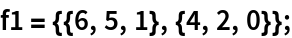This finds the partition corresponding to the Frobenius symbol:

 In:=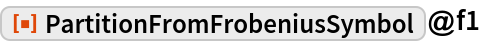Out=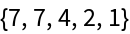Here is another example:

 In:=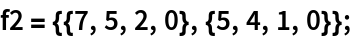In:=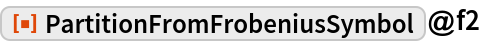Out=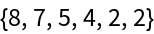### Properties and Relations

Confirm that partitions and Frobenius symbols round-trip as expected:

 In:=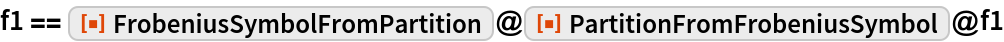Out=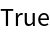In:=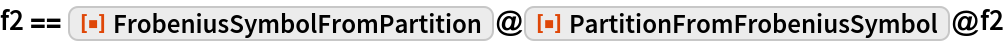Out=